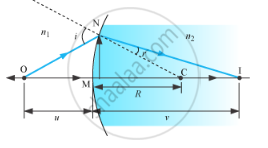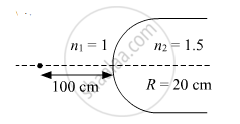Share

# Derive the Mathematical Relation Between Refractive Indices N1 and N2 of Two Radii and Radius of Curvature R for Refraction at a Convex Spherical Surface. - CBSE (Science) Class 12 - Physics

#### Question

(i) Derive the mathematical relation between refractive indices n1 and n2 of two radii and radius of curvature R for refraction at a convex spherical surface. Consider the object to be a point since lying on the principle axis in rarer medium of refractive index n1 and a real image formed in the denser medium of refractive index n2. Hence, derive lens maker's formula.

(ii) Light from a point source in air falls on a convex spherical glass surface of refractive index 1.5 and radius of curvature 20 cm. The distance of light source from the glass surface is 100 cm. At what position is the image formed?

#### Solution

(i)The above figure shows the geometry of formation of real image I of an object O and the principal axis of a spherical surface with centre of curvature C and radius of curvature R

Assumptions:

(i) The aperture of the surface is small compared to other distance involved.

(ii) NM will be taken to be nearly equal to the length of the perpendicular from the point N on the principal axis.

tan /_NOM=(MN)/(OM)

tan/_NCM=(MN)/(MC)

tan /_NIM=(MN)/(MI)

For ΔNOC, i is the exterior angle.
Assuming the incident ray is very close to the principal axis, all the angles are very small. Hence, for very small angles

tan x = x = sin x

:.i=/_NOM+/_NCM

i=(MN)/(OM)+(MN)/(MC)" .....(i)"

Similarly, r = ∠NCM − ∠NIM

i.e,r=(MN)/(MC)-(MN)/(MI)" ...(ii) "

By Snell’s law,

n1sini = n2sinr

For small angles

n1in2 r

Substituting the values of i and r from equations (i) and (ii), we obtain

n_1((MN)/(OM)+(MN)/(MC))=n_2((MN)/(MC)-(MN)/(MI))

Or,n_1/(OM)+n_2/(MI)=(n_2-n_1)/(MC)" ....(iii)"

Applying new Cartesian sign conventions,

OM = − u, MI = + v, MC = + R

Substituting these in equation (iii), we obtain

 n_2/v-n_1/u=(n_2-n_1)/R

...(iV)

(ii)n_2/v-n_1/u=(n_2-n_1)/R

=>1.5/V-1/((-100))=(1.5-1)/20=1/40

=>1.5/V=1/40-1/100=(5-2)/200=3/200

=>v=(1.5xx200)/3=100 cm

Hence, the image is formed 100 cm in the denser medium

Is there an error in this question or solution?

#### Video TutorialsVIEW ALL 

Solution Derive the Mathematical Relation Between Refractive Indices N1 and N2 of Two Radii and Radius of Curvature R for Refraction at a Convex Spherical Surface. Concept: Lensmaker's Formula.
S